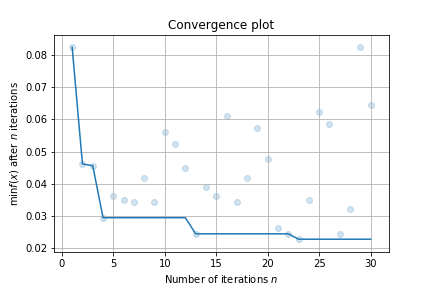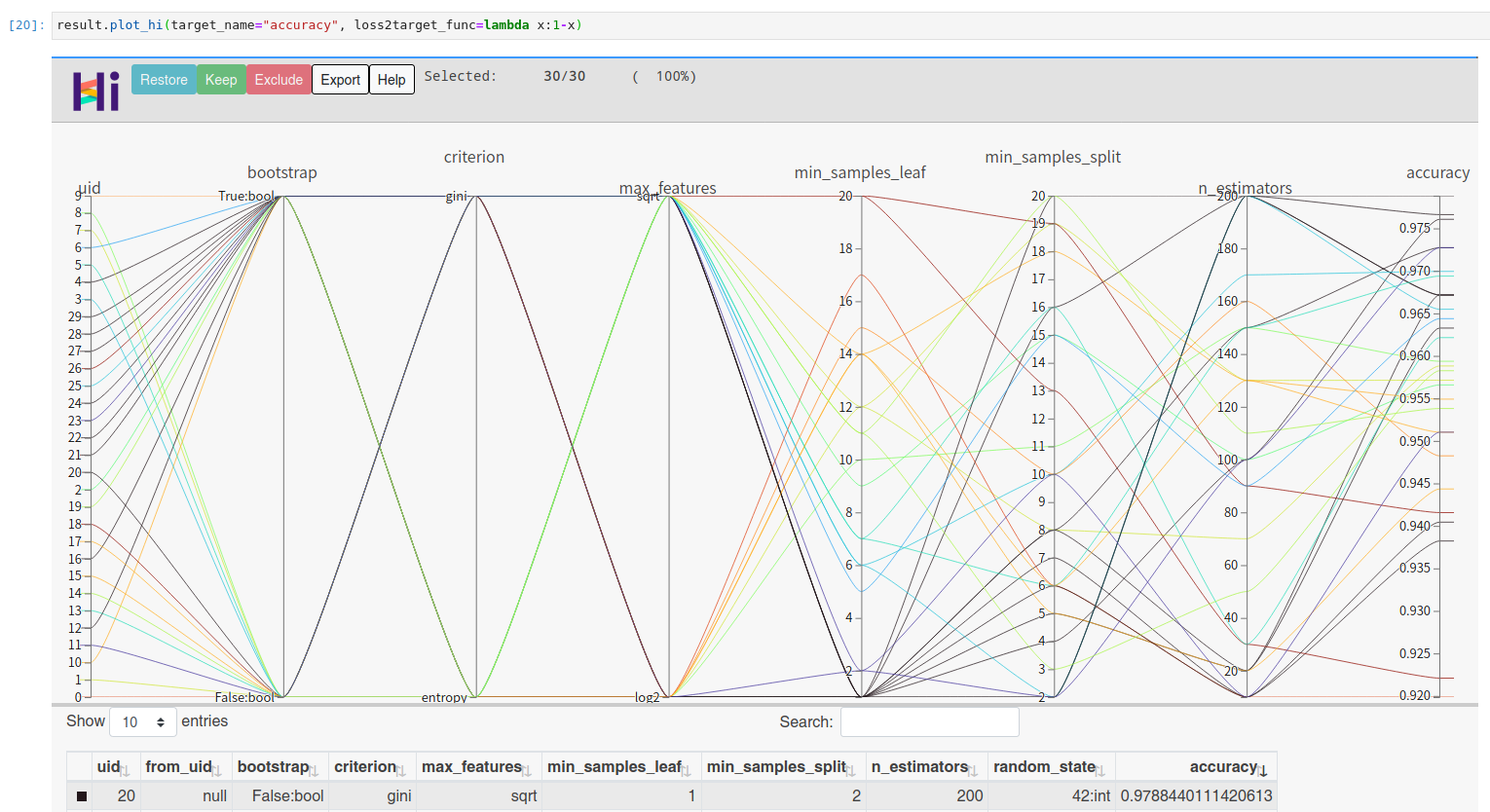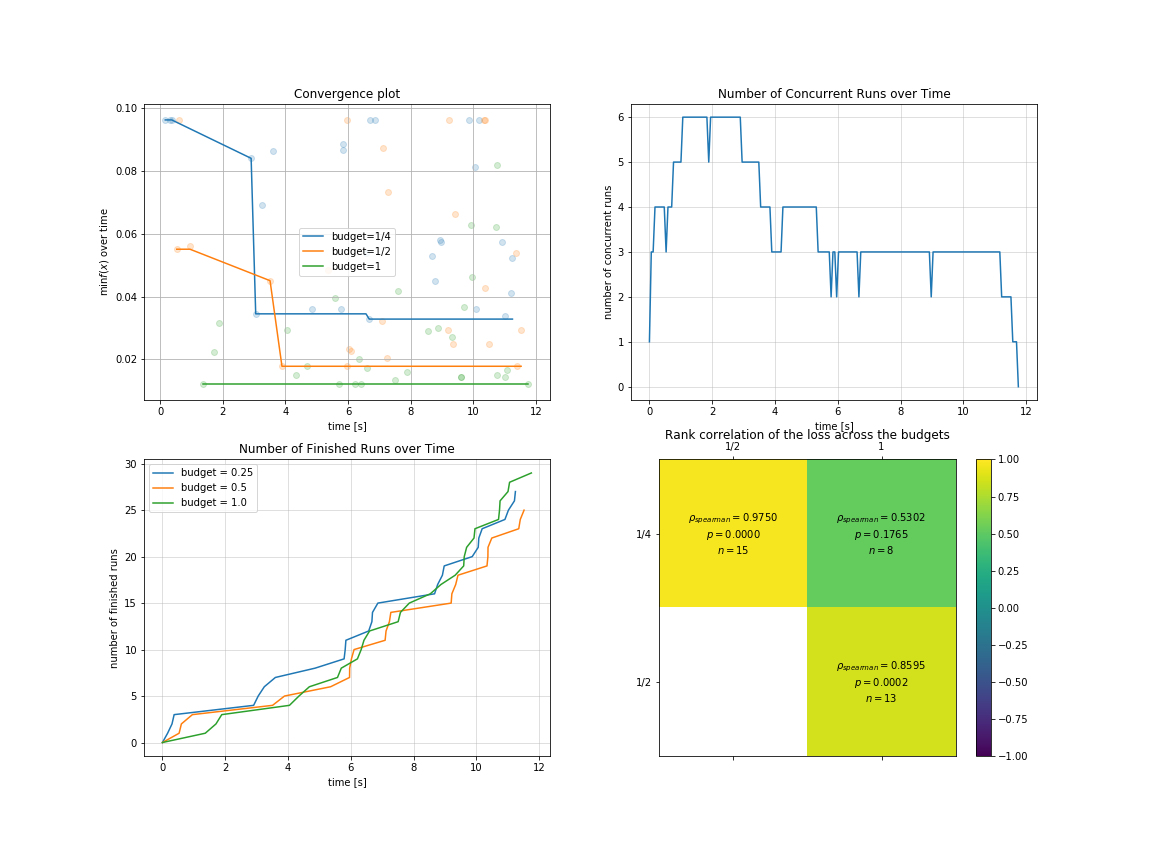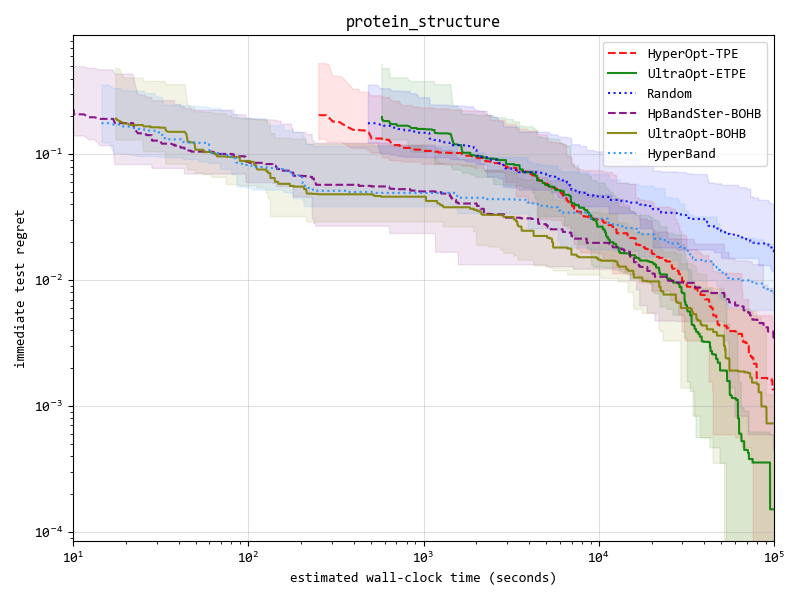## UltraOpt

UltraOpt is a simple and efficient library to minimize expensive and noisy black-box functions, it can be used in many fields, such as HyperParameter Optimization(HPO) and Automatic Machine Learning(AutoML).

After absorbing the advantages of existing optimization libraries such as HyperOpt, SMAC3, scikit-optimize and HpBandSter, we develop UltraOpt , which implement a new bayesian optimization algorithm : Embedding-Tree-Parzen-Estimator(ETPE), which is better than HyperOpt' TPE algorithm in our experiments. Besides, The optimizer of UltraOpt is redesigned to adapt HyperBand & SuccessiveHalving Evaluation Strategies and MapReduce & Async Communication Conditions. Finally, you can visualize Config Space and optimization process & results by UltraOpt's tool function. Enjoy it !

# Installation

UltraOpt requires Python 3.6 or higher.

You can install the latest release by `pip`:

``````pip install ultraopt
``````

``````git clone https://github.com/auto-flow/ultraopt.git && cd ultraopt
python setup.py install
``````

# Quick Start

## Using UltraOpt in HPO

Let's learn what `UltraOpt` doing with several examples (you can try it on your `Jupyter Notebook`).

You can learn Basic-Tutorial in here, and `HDL`'s Definition in here.

Before starting a black box optimization task, you need to provide two things:

• parameter domain, or the Config Space
• objective function, accept `config` (`config` is sampled from Config Space), return `loss`

Let's define a Random Forest's HPO Config Space by `UltraOpt`'s `HDL` (Hyperparameter Description Language):

``````HDL = {
"n_estimators": {"_type": "int_quniform","_value": [10, 200, 10], "_default": 100},
"criterion": {"_type": "choice","_value": ["gini", "entropy"],"_default": "gini"},
"max_features": {"_type": "choice","_value": ["sqrt","log2"],"_default": "sqrt"},
"min_samples_split": {"_type": "int_uniform", "_value": [2, 20],"_default": 2},
"min_samples_leaf": {"_type": "int_uniform", "_value": [1, 20],"_default": 1},
"bootstrap": {"_type": "choice","_value": [True, False],"_default": True},
"random_state": 42
}
``````

And then define an objective function:

``````from sklearn.ensemble import RandomForestClassifier
from sklearn.model_selection import cross_val_score, StratifiedKFold
from ultraopt.hdl import layering_config
cv = StratifiedKFold(5, True, 0)
def evaluate(config: dict) -> float:
model = RandomForestClassifier(**layering_config(config))
return 1 - float(cross_val_score(model, X, y, cv=cv).mean())
``````

Now, we can start an optimization process:

``````from ultraopt import fmin
result = fmin(eval_func=evaluate, config_space=HDL, optimizer="ETPE", n_iterations=30)
result
``````
``````100%|██████████| 30/30 [00:36<00:00,  1.23s/trial, best loss: 0.023]

+-----------------------------------+
| HyperParameters   | Optimal Value |
+-------------------+---------------+
| bootstrap         | True:bool     |
| criterion         | gini          |
| max_features      | log2          |
| min_samples_leaf  | 1             |
| min_samples_split | 2             |
| n_estimators      | 200           |
+-------------------+---------------+
| Optimal Loss      | 0.0228        |
+-------------------+---------------+
| Num Configs       | 30            |
+-------------------+---------------+
``````

Finally, make a simple visualizaiton:

``````result.plot_convergence()
``````You can visualize high dimensional interaction by facebook's hiplot:

``````!pip install hiplot
result.plot_hi(target_name="accuracy", loss2target_func=lambda x:1-x)
``````## Using UltraOpt in AutoML

Let's try a more complex example: solve AutoML's CASH Problem  (Combination problem of Algorithm Selection and Hyperparameter optimization)
by BOHB algorithm (Combine HyperBand Evaluation Strategies with `UltraOpt`'s ETPE optimizer) .

You can learn Conditional Parameter and complex `HDL`'s Definition in here, AutoML implementation tutorial in here and Multi-Fidelity Optimization in here.

First of all, let's define a CASH `HDL` :

``````HDL = {
'classifier(choice)':{
"RandomForestClassifier": {
"n_estimators": {"_type": "int_quniform","_value": [10, 200, 10], "_default": 100},
"criterion": {"_type": "choice","_value": ["gini", "entropy"],"_default": "gini"},
"max_features": {"_type": "choice","_value": ["sqrt","log2"],"_default": "sqrt"},
"min_samples_split": {"_type": "int_uniform", "_value": [2, 20],"_default": 2},
"min_samples_leaf": {"_type": "int_uniform", "_value": [1, 20],"_default": 1},
"bootstrap": {"_type": "choice","_value": [True, False],"_default": True},
"random_state": 42
},
"KNeighborsClassifier": {
"n_neighbors": {"_type": "int_loguniform", "_value": [1,100],"_default": 3},
"weights" : {"_type": "choice", "_value": ["uniform", "distance"],"_default": "uniform"},
"p": {"_type": "choice", "_value": [1, 2],"_default": 2},
},
}
}
``````

And then, define a objective function with an additional parameter `budget` to adapt to HyperBand evaluation strategy:

``````from sklearn.neighbors import KNeighborsClassifier
import numpy as np
def evaluate(config: dict, budget: float) -> float:
layered_dict = layering_config(config)
AS_HP = layered_dict['classifier'].copy()
AS, HP = AS_HP.popitem()
ML_model = eval(AS)(**HP)
scores = []
for i, (train_ix, valid_ix) in enumerate(cv.split(X, y)):
rng = np.random.RandomState(i)
size = int(train_ix.size * budget)
train_ix = rng.choice(train_ix, size, replace=False)
X_train,y_train = X[train_ix, :],y[train_ix]
X_valid,y_valid = X[valid_ix, :],y[valid_ix]
ML_model.fit(X_train, y_train)
scores.append(ML_model.score(X_valid, y_valid))
score = np.mean(scores)
return 1 - score
``````

You should instance a `multi_fidelity_iter_generator` object for the purpose of using HyperBand Evaluation Strategy :

``````from ultraopt.multi_fidelity import HyperBandIterGenerator
hb = HyperBandIterGenerator(min_budget=1/4, max_budget=1, eta=2)
hb.get_table()
``````
iter 0 iter 1 iter 2
stage 0 stage 1 stage 2 stage 0 stage 1 stage 0
num_config 4 2 1 2 1 3
budget 1/4 1/2 1 1/2 1 1

let's combine HyperBand Evaluation Strategies with `UltraOpt`'s ETPE optimizer , and then start an optimization process:

``````result = fmin(eval_func=evaluate, config_space=HDL,
optimizer="ETPE", # using bayesian optimizer: ETPE
multi_fidelity_iter_generator=hb, # using HyperBand
n_iterations=20)
result
``````
``````100%|██████████| 88/88 [00:11<00:00,  7.48trial/s, max budget: 1.0, best loss: 0.012]

+--------------------------------------------------------------------------------------------------------------------------+
| HyperParameters                                     | Optimal Value                                                      |
+-----------------------------------------------------+----------------------+----------------------+----------------------+
| classifier:__choice__                               | KNeighborsClassifier | KNeighborsClassifier | KNeighborsClassifier |
| classifier:KNeighborsClassifier:n_neighbors         | 4                    | 1                    | 3                    |
| classifier:KNeighborsClassifier:p                   | 2:int                | 2:int                | 2:int                |
| classifier:KNeighborsClassifier:weights             | distance             | uniform              | uniform              |
| classifier:RandomForestClassifier:bootstrap         | -                    | -                    | -                    |
| classifier:RandomForestClassifier:criterion         | -                    | -                    | -                    |
| classifier:RandomForestClassifier:max_features      | -                    | -                    | -                    |
| classifier:RandomForestClassifier:min_samples_leaf  | -                    | -                    | -                    |
| classifier:RandomForestClassifier:min_samples_split | -                    | -                    | -                    |
| classifier:RandomForestClassifier:n_estimators      | -                    | -                    | -                    |
| classifier:RandomForestClassifier:random_state      | -                    | -                    | -                    |
+-----------------------------------------------------+----------------------+----------------------+----------------------+
| Budgets                                             | 1/4                  | 1/2                  | 1 (max)              |
+-----------------------------------------------------+----------------------+----------------------+----------------------+
| Optimal Loss                                        | 0.0328               | 0.0178               | 0.0122               |
+-----------------------------------------------------+----------------------+----------------------+----------------------+
| Num Configs                                         | 28                   | 28                   | 32                   |
+-----------------------------------------------------+----------------------+----------------------+----------------------+
``````

You can visualize optimization process in `multi-fidelity` scenarios:

``````import pylab as plt
plt.rcParams['figure.figsize'] = (16, 12)
plt.subplot(2, 2, 1)
result.plot_convergence_over_time();
plt.subplot(2, 2, 2)
result.plot_concurrent_over_time(num_points=200);
plt.subplot(2, 2, 3)
result.plot_finished_over_time();
plt.subplot(2, 2, 4)
result.plot_correlation_across_budgets();
``````## Advantage One: ETPE optimizer is more competitive

We implement 4 kinds of optimizers(listed in the table below), and `ETPE` optimizer is our original creation, which is proved to be better than other `TPE based optimizers` such as `HyperOpt's TPE` and `HpBandSter's BOHB` in our experiments.

Our experimental code is public available in here, experimental documentation can be found in here .

Optimizer Description
ETPE Embedding-Tree-Parzen-Estimator, is our original creation, converting high-cardinality categorical variables to low-dimension continuous variables based on TPE algorithm, and some other aspects have also been improved, is proved to be better than `HyperOpt's TPE` in our experiments.
Forest Bayesian Optimization based on Random Forest. Surrogate model import `scikit-optimize` 's `skopt.learning.forest` model, and integrate Local Search methods in `SMAC3`
GBRT Bayesian Optimization based on Gradient Boosting Resgression Tree. Surrogate model import `scikit-optimize` 's `skopt.learning.gbrt` model.
Random Random Search for baseline or dummy model.

Key result figure in experiment (you can see details in experimental documentation ) :You can see this section in the documentation:

## Advantage Three: UltraOpt is more function comlete and user friendly

UltraOpt is more function comlete and user friendly than other optimize library:

UltraOpt HyperOpt Scikit-Optimize SMAC3 HpBandSter
Simple Usage like `fmin` function ×
Simple `Config Space` Definition × ×
Support Conditional `Config Space` ×
Support Serializable `Config Space` × × × ×
Support Visualizing `Config Space` × × ×
Can Analyse Optimization Process & Result × ×
Distributed in Cluster × ×
Support HyperBand & SuccessiveHalving × ×

# Citation

``````@misc{Tang_UltraOpt,
author       = {Qichun Tang},
title        = {UltraOpt : Distributed Asynchronous Hyperparameter Optimization better than HyperOpt},
month        = January,
year         = 2021,
doi          = {10.5281/zenodo.4430148},
version      = {v0.1.0},
publisher    = {Zenodo},
url          = {https://doi.org/10.5281/zenodo.4430148}
}
``````